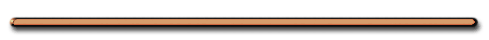HomeVisit Show Me The Physics's YouTube Channel

Video Instruction

showmethephysics.com

G. Equilibrium - resultant = 0 NEx 1)At Equilibrium:Fnet = 0, a = 0Velocity is Constant

(rest or constant v)

Ex 2) Could the forces of 8 N, 6 N and 4 N ever produce a state of equilibrium?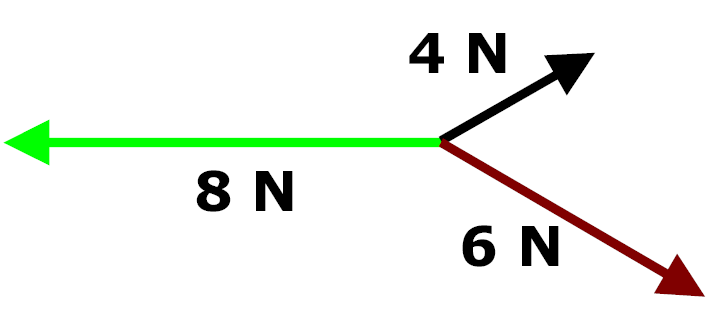If so, find the resultant of the forces 4 N and 6 N.

Yes, resultant of the forces 4 and 6 would have to be 8 N

Ex 3) Could the angles of these forces ever be arranged to produce equilibrium?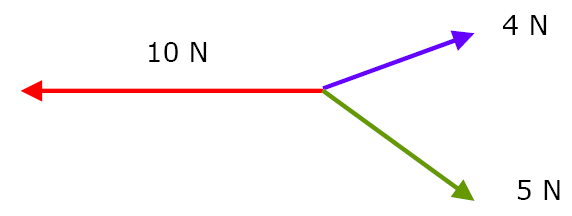NO

4 N and 5 N have a range of possible
resultants of 1 N and 9 NCould these forces ever
be in equilibrium?No,

50 N & 15 N have a
range of 65 N - 35 NWhich diagram   represents a box in  equilibrium?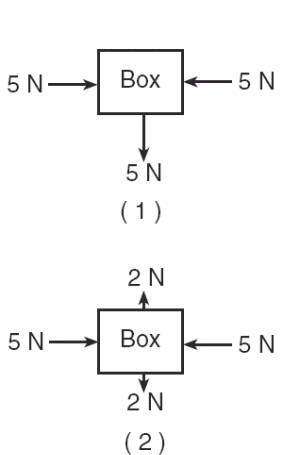June 2008 #9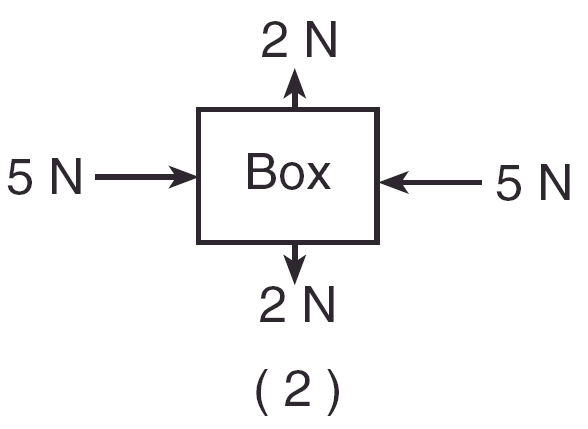RankingTaskRank the resultants from smallest to largest.